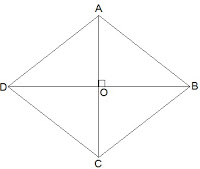Guru

# Show that if the diagonals of a quadrilateral bisect each other at right angles, then it is a rhombus. Q.3

• 0

I want to know the best answer of the question from Quadrilaterals chapter of class 9th ncert math. The question from exercise 8.1of math. Give me the easy way for solving this question of 2 Show that if the diagonals of a quadrilateral bisect each other at right angles, then it is a rhombus

Share

1.Let ABCD be a quadrilateral whose diagonals bisect each other at right angles.

Given that,

OA = OC

OB = OD

and ∠AOB = ∠BOC = ∠OCD = ∠ODA = 90°

To show that,

if the diagonals of a quadrilateral bisect each other at right angles, then it is a rhombus.

i.e., we have to prove that ABCD is parallelogram and AB = BC = CD = AD

Proof,

In ΔAOB and ΔCOB,

OA = OC (Given)

∠AOB = ∠COB (Opposite sides of a parallelogram are equal)

OB = OB (Common)

Therefore, ΔAOB ≅ ΔCOB [SAS congruency]

Thus, AB = BC [CPCT]

Similarly we can prove,

BC = CD